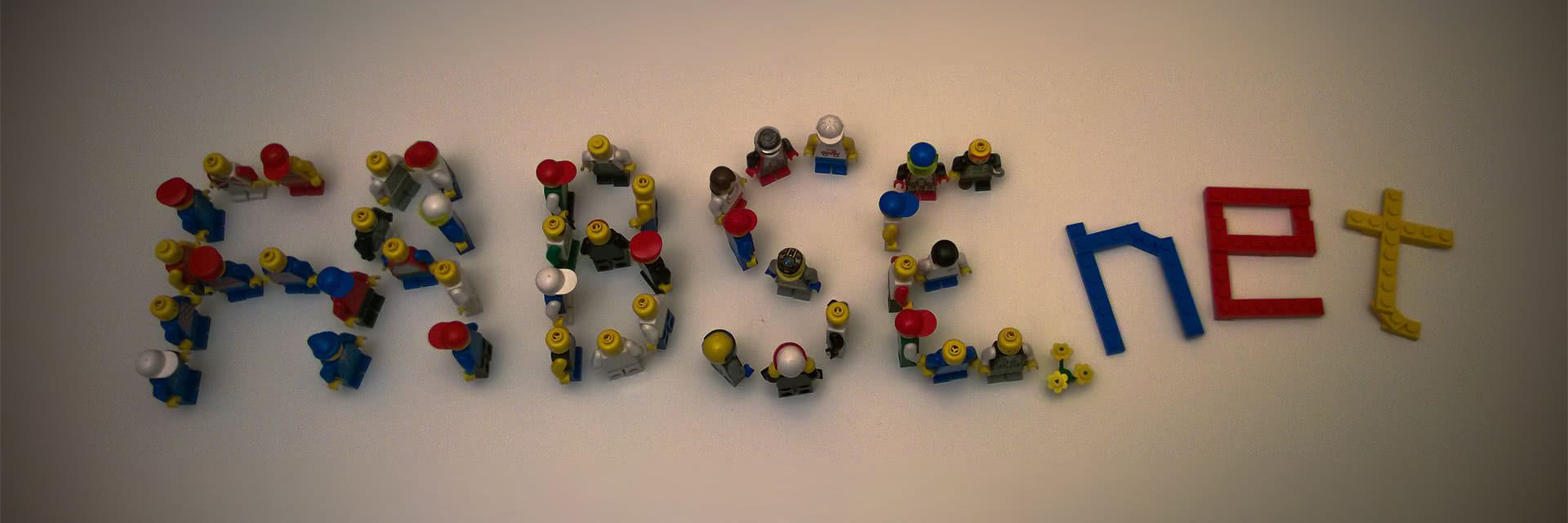• Fabian Wetzel
• 2014-12-10

# Linq: Finding the inverse operation to SelectMany()

I am searching for the inverse operation to the SelectMany() linq method. But at first, we have to understand the SelectMany() method. Consider a simple array containing arrays of numbers and we do SelectMany() on them:

This will output the single list of numbers:

For the inverse operation, we have this single list of numbers and are trying to put them in different lists to build the given groups above again. Obviously we need some kind of way to decide, whether a given number should be added to the current list or should produce a new list. To solve this, we could do the integer division by 10 in a group by on all numbers, like so:

This outputs exactly what I wanted:

The way my actual task works is that I only know whether a given number belongs to the list or should start a new list by looking at the previous number. We could express this as the following predicate:

The signature of the method is important as it needs not only the current item but also the previous item of the list! This is the point where GroupBy() is of no help anymore and where I got stuck for some time.

The Linq method I am searching should have a signature to something like this:

Knowing the correct target is 90% of the solution. So having found the signature, I came up with the following solution:

This will build up an internal list of items as long as they belong together and will yield the list, if they do not belong together anymore.

The usage looks like:

This does now exactly what I needed :-)